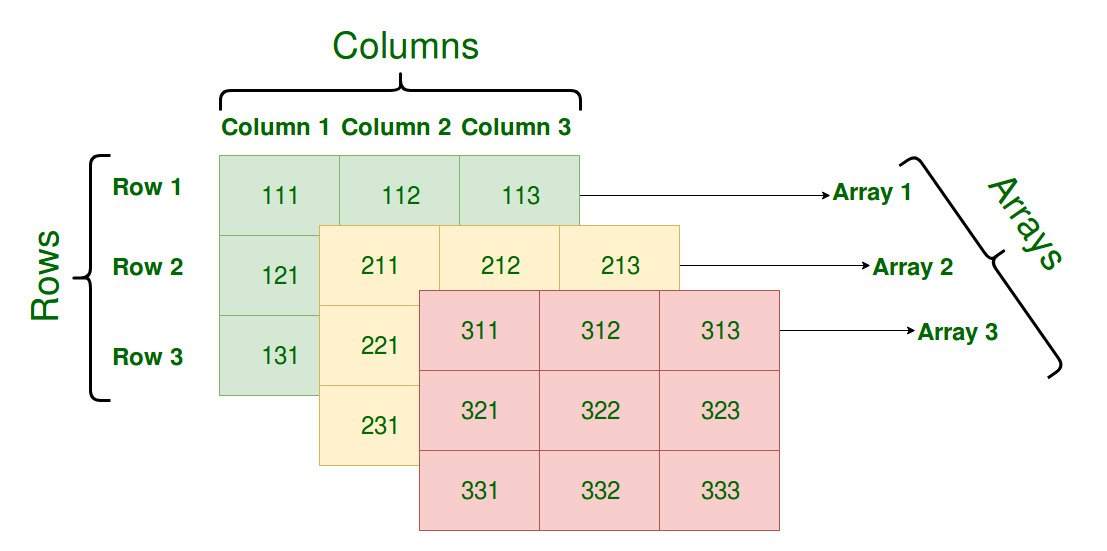# Multidimensional arrays in PHP

Multi-dimensional arrays are such type of arrays which stores an another array at each index instead of single element. In other words, define multi-dimensional arrays as array of arrays. As the name suggests, every element in this array can be an array and they can also hold other sub-arrays within. Arrays or sub-arrays in multidimensional arrays can be accessed using multiple dimensions.

Dimensions: Dimensions of multidimensional array indicates the number of indices needed to select an element. For a two dimensional array two indices to select an element.

Two dimensional array: It is the simplest form of a multidimensional array. It can be created using nested array. These type of arrays can be used to store any type of elements, but the index is always a number. By default, the index starts with zero.

Syntax:

```array (
array (elements...),
array (elements...),
...
)```Example:

 ` `

Output:

```Array
(
 => Array
(
 => Ankit
 => Ram
 => Shyam
)

 => Array
(
 => Unnao
 => Trichy
 => Kanpur
)

)
```

Two dimensional associative array: Al associative array is similar to indexed array but instead of linear storage (indexed storage), every value can be assigned with a user-defined key of string type.

Example:

 ` ``array``( ` `         `  `        ``// Subject and marks are ` `        ``// the key value pair ` `        ``"C"` `=> 95, ` `        ``"DCO"` `=> 85, ` `        ``"FOL"` `=> 74, ` `    ``), ` `         `  `    ``// Ram will act as key ` `    ``"Ram"` `=> ``array``( ` `         `  `        ``// Subject and marks are ` `        ``// the key value pair ` `        ``"C"` `=> 78, ` `        ``"DCO"` `=> 98, ` `        ``"FOL"` `=> 46, ` `    ``), ` `     `  `    ``// Anoop will act as key ` `    ``"Anoop"` `=> ``array``( ` `         `  `        ``// Subject and marks are ` `        ``// the key value pair ` `        ``"C"` `=> 88, ` `        ``"DCO"` `=> 46, ` `        ``"FOL"` `=> 99, ` `    ``), ` `); ` ` `  `echo` `"Display Marks: \n"``; ` `     `  `print_r(``\$marks``); ` `?> `

Output:

```Display Marks:
Array
(
[Ankit] => Array
(
[C] => 95
[DCO] => 85
[FOL] => 74
)

[Ram] => Array
(
[C] => 78
[DCO] => 98
[FOL] => 46
)

[Anoop] => Array
(
[C] => 88
[DCO] => 46
[FOL] => 99
)

)
```

Three Dimensional Array: It is the form of multidimensional array. Initialization in Three-Dimensional array is same as that of Two-dimensional arrays. The difference is as the number of dimension increases so the number of nested braces will also increase.

Syntax:

```array (
array (
array (elements...),
array (elements...),
...
),
array (
array (elements...),
array (elements...),
...
),
...
)```Example:

 ` `

Output:

```Array
(
 => Array
(
 => Array
(
 => 1
 => 2
)

 => Array
(
 => 3
 => 4
)

)

 => Array
(
 => Array
(
 => 5
 => 6
)

 => Array
(
 => 7
 => 8
)

)

)
```

Accessing multidimensional array elements: There are mainly two ways to access multidimensional array elements in PHP.

• Elements can be accessed using dimensions as array_name[‘first dimension’][‘second dimension’].
• Elements can be accessed using for loop.
• Elements can be accessed using for each loop.

Example:

 ` ``array``( ` `         `  `        ``// Subject and marks are  ` `        ``// the key value pair ` `        ``"C"` `=> 95, ` `        ``"DCO"` `=> 85, ` `        ``"FOL"` `=> 74, ` `    ``), ` `         `  `    ``// Ram will act as key ` `    ``"Ram"` `=> ``array``( ` `         `  `        ``// Subject and marks are  ` `        ``// the key value pair ` `        ``"C"` `=> 78, ` `        ``"DCO"` `=> 98, ` `        ``"FOL"` `=> 46, ` `    ``), ` `     `  `    ``// Anoop will act as key ` `    ``"Anoop"` `=> ``array``( ` `         `  `        ``// Subject and marks are ` `        ``// the key value pair ` `        ``"C"` `=> 88, ` `        ``"DCO"` `=> 46, ` `        ``"FOL"` `=> 99, ` `    ``), ` `); ` ` `  `// Accessing the array element  ` `// using dimensions  ` `     `  `// It will display the marks of ` `// Ankit in C subject ` `echo` `\$marks``[``'Ankit'``][``'C'``] . ``"\n"``;  ` `     `  `// Accessing array elements using for each loop ` `foreach``(``\$marks` `as` `\$mark``) { ` `    ``echo` `\$mark``[``'C'``]. ``" "``.``\$mark``[``'DCO'``].``" "``.``\$mark``[``'FOL'``].``"\n"``;  ` `} ` `     `  `?> `

Output:

```95
95 85 74
78 98 46
88 46 99
```

My Personal Notes arrow_drop_upCheck out this Author's contributed articles.

If you like GeeksforGeeks and would like to contribute, you can also write an article using contribute.geeksforgeeks.org or mail your article to contribute@geeksforgeeks.org. See your article appearing on the GeeksforGeeks main page and help other Geeks.

Please Improve this article if you find anything incorrect by clicking on the "Improve Article" button below.